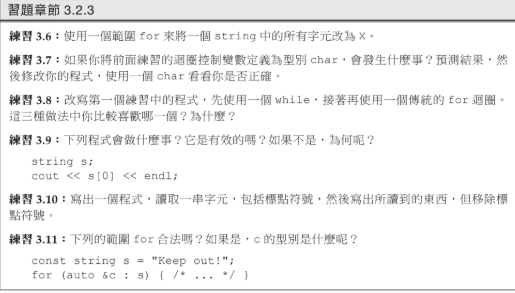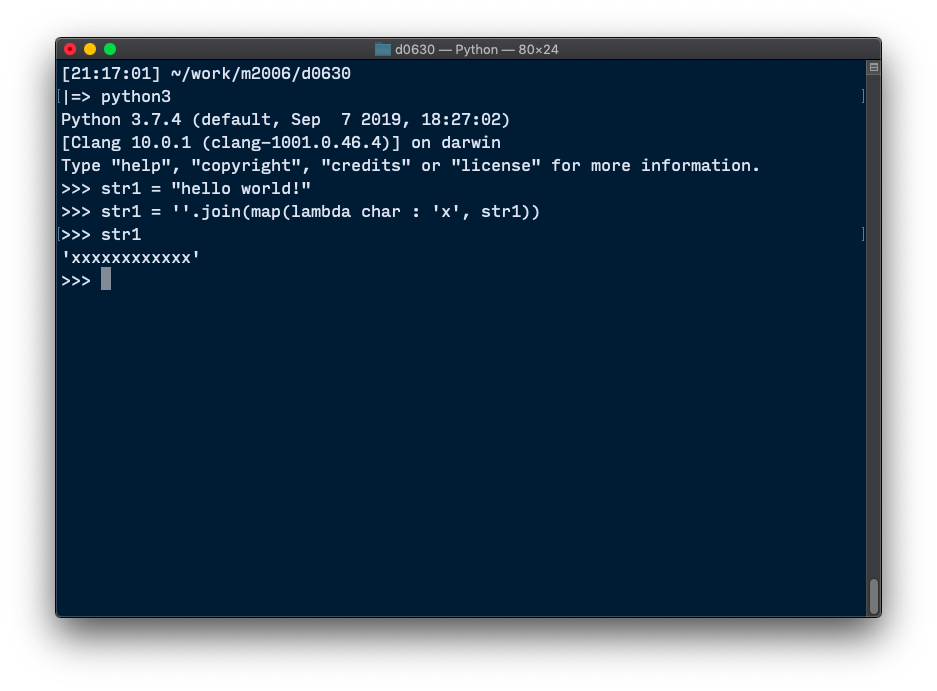#1

## String的問題### 4 個回答

4

https://github.com/huangmingchuan/Cpp_Primer_Answers/blob/master/ch03/exercise3_6.cpp

https://github.com/huangmingchuan/Cpp_Primer_Answers

https://sanmin.com.tw/Product/index/004969836

``````str1 = "hello world!"
str1 = ''.join(map(lambda char : 'x', str1))
``````froce iT邦大師 1 級 ‧ 2020-07-01 08:21:49 檢舉
``````str1 = "hello world!"
str1 = ["x" for i in str1]
``````

``````str1 = "hello world!"
str1 = 'x' * len(str1)
``````

froce iT邦大師 1 級 ‧ 2020-07-01 09:41:56 檢舉

3
``````#include <iostream>
#include <string>

int main()
{
std::string a ("Andrew Choi");
for (int i = 0; i < a.size(); ++i)
{
a[i] = 'x';
}

std::cout << a ;
}

``````

5``````#include <iostream>

using namespace std;

int main()
{
string str = "Hello World";
string ans = "";

for (int i=0;i<str.length();i++) {
ans = ans + 'x';
}
cout << ans << endl;

return 0;
}
``````
0
``````let string = '123456789';
console.log(string.replace(/\w/g, 'X'));
``````